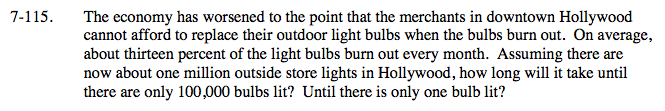### Home > A2C > Chapter 7 > Lesson 7.2.2 > Problem7-115

7-115.Set up an exponential equation to represent this situation.

y = 1,000,000(0.87)x

Let y = 100,000:

100,000 = 1,000,000(0.87)x

To solve, divide both sides of the equation by 1,000,000. Then take the log of both sides and apply the power property.